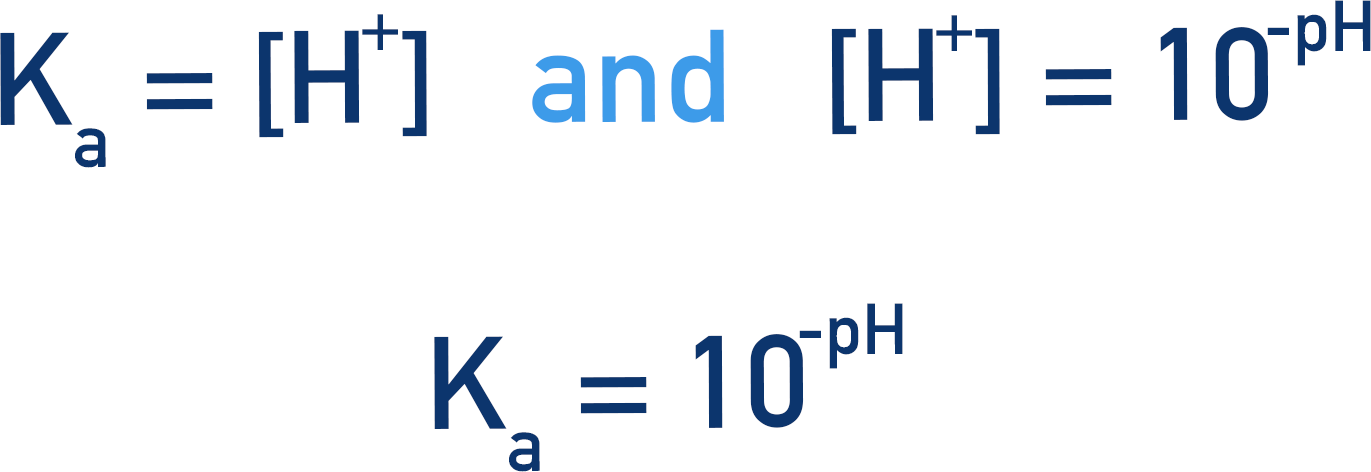Video Tutorial Finding Ka from Titration Curve

Quick Notes Finding Ka from Titration Curve

• The acid dissociation constant (Ka) for a weak acid (HA) can be found using a titration curve.
• At the equivalence point, the concentration of HA is zero and the concentration of A- will be the same as the original concentration of HA.
• At the half-equivalence point, the concentrations of HA and A- will be the same.
• In the Ka expression for a weak acid, HA and A- cancel each other out. Ka will be the same as the concentration of H+ ions.
• This means Ka = 10-pH

Full Notes Finding Ka from Titration Curve

The Ka value for a weak acid can be found by using a titration curve.

At the equivalence point, the exact amount of base has been added to react completely with all the acid (HA) that was present in the solution. The concentration of acid in the solution is now zero and the concentration of base is also zero.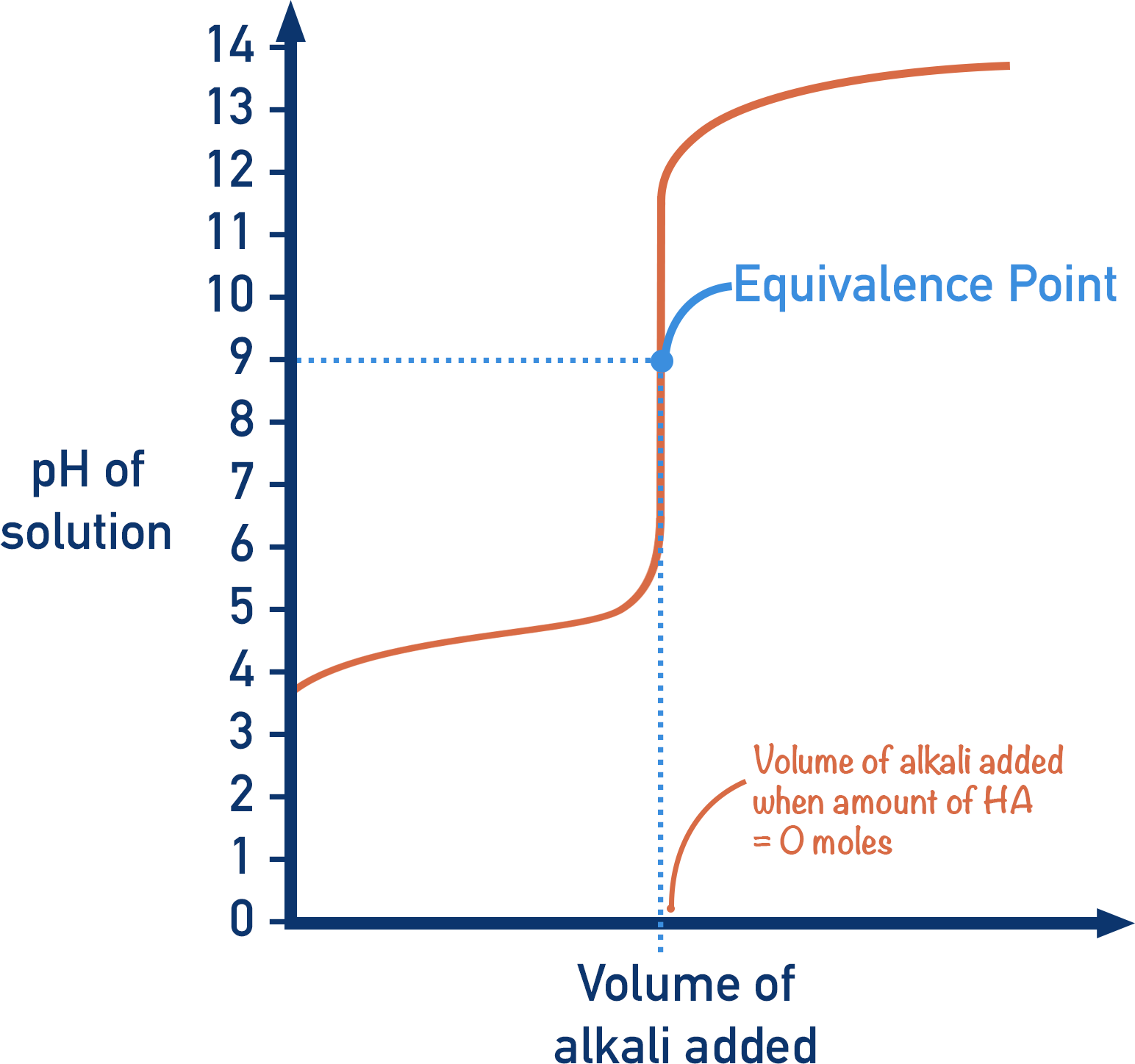The important thing is to recognise that when the concentration of acid (HA) is zero, the concentration of A-(aq) will be the same as the original concentration of acid. This is because A-(aq) is formed when the HA(aq) reacts with OH-(aq).When half the HA(aq) has reacted with OH-(aq), the concentrations of HA(aq) and A-(aq) will be the same. This is the ‘half equivalence’ point.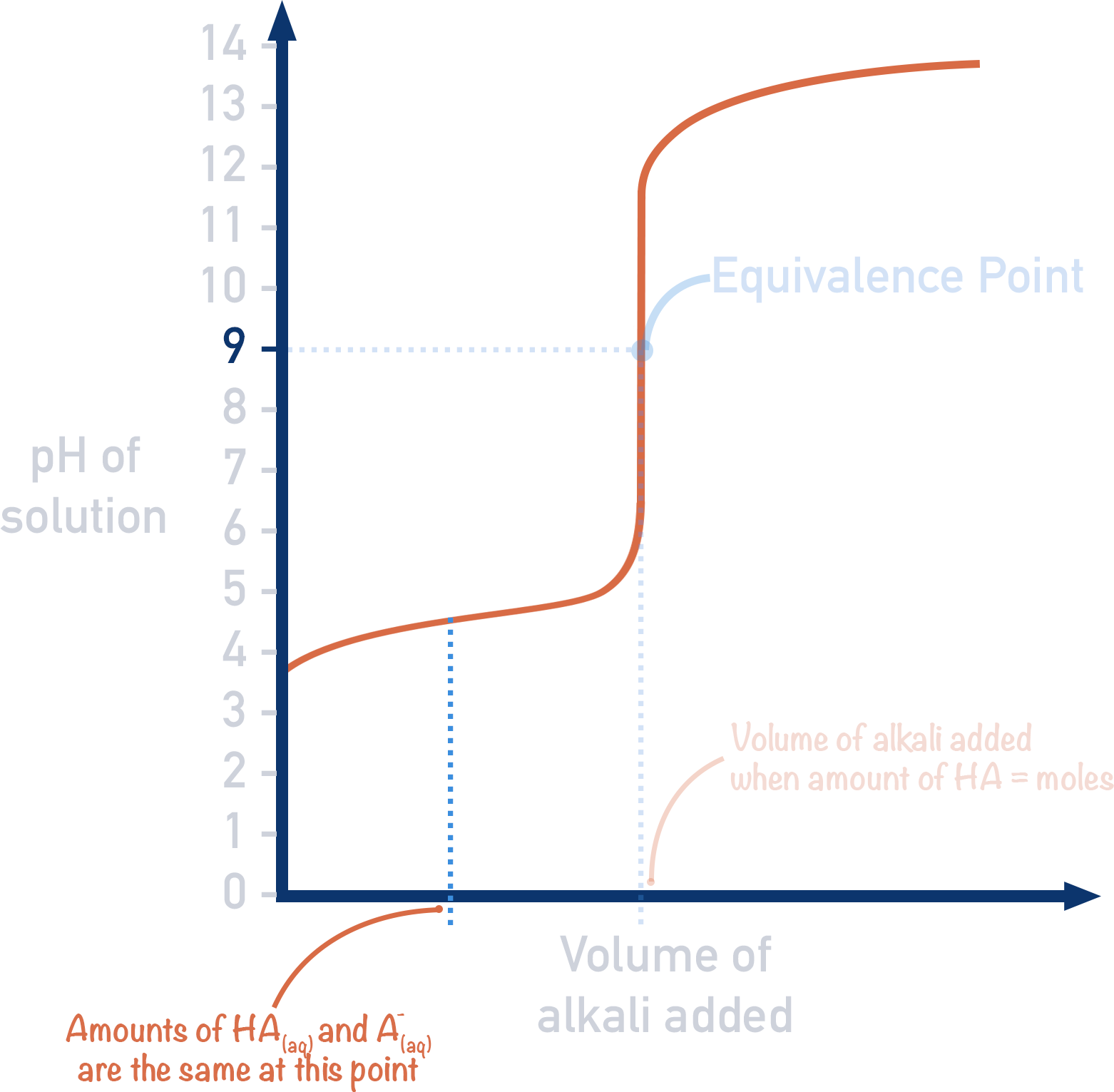When we look at the expression for Ka, we can see that if the concentrations of HA(aq) and A-(aq) are the same, they cancel each other.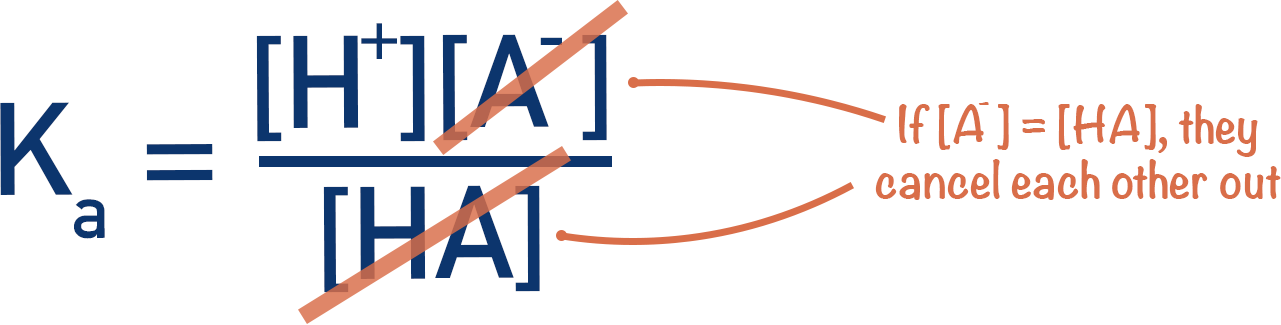The expression can now be simplified.The concentration of H+(aq) ions can be found by taking the pH of the solution when the concentrations of HA(aq) and A-(aq) are the same (half equivalence point).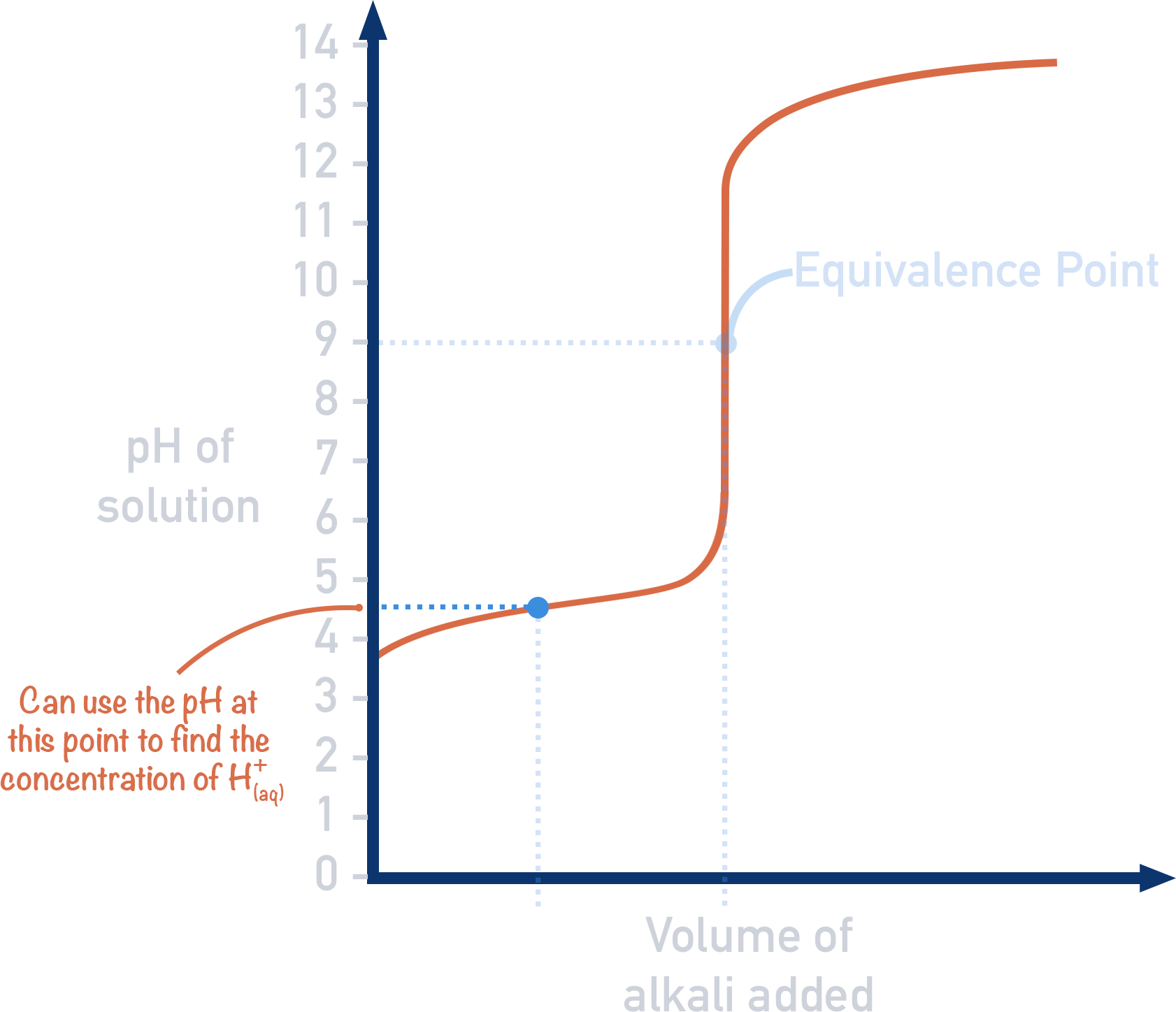We can find the concentration of H+(aq) ions using: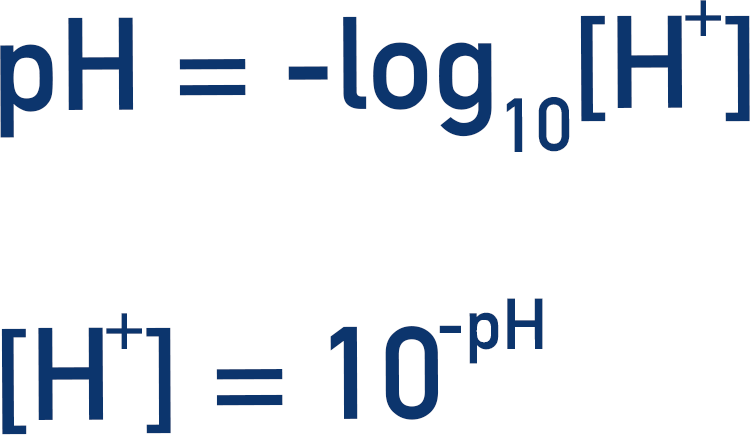Substituting the two equations gives: